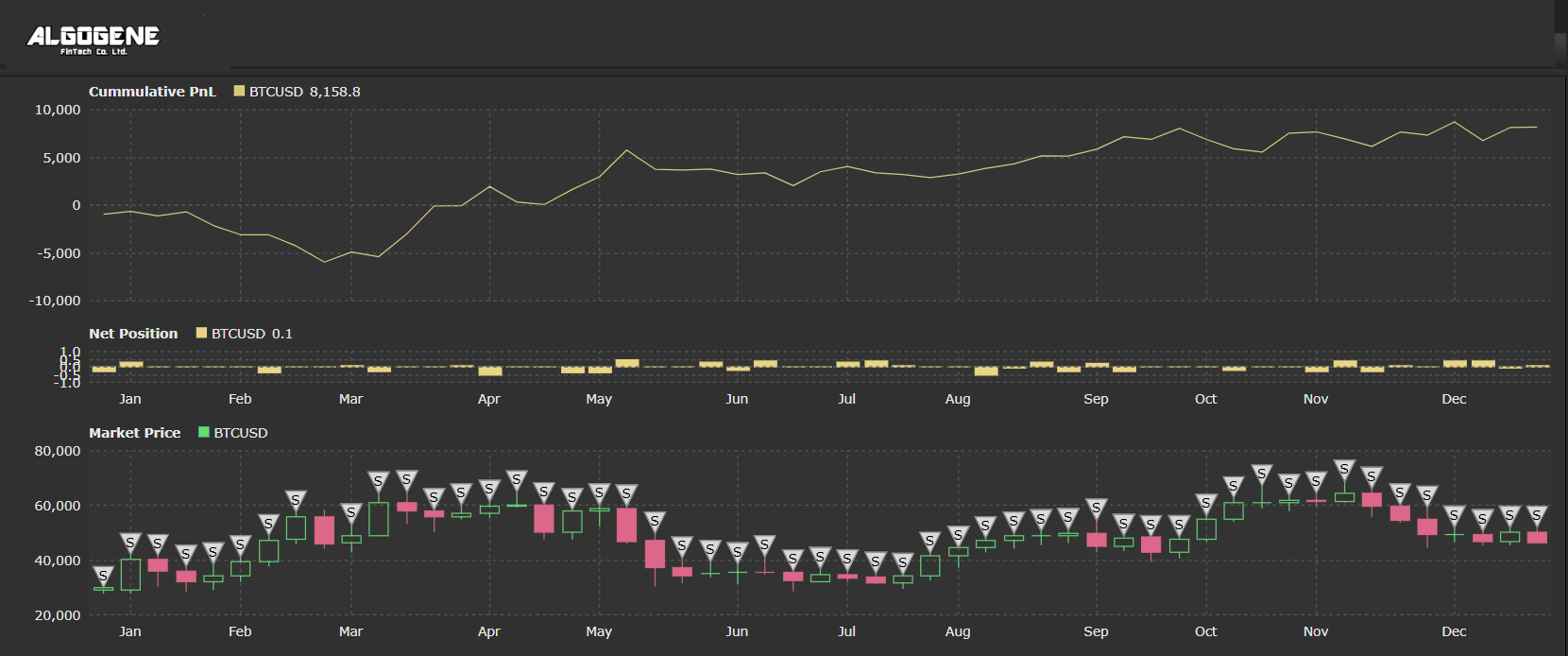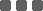## Implement Grid Trading Strategy in Python

Hello everyone! This post is all about implementing a Grid Trading strategy on ALGOGENE. If you are interested to learn how this strategy works or if you are a new joiner of the platform, this post is for you!

A Grid Trading Strategy places a set of buy and sell orders in regular intervals above and below the current price. As the price ranges up and down, long and short orders are being executed and will generate a profit as the price continues to move up and down the grid.

There are 2 versions of grid strategy for

• Ranging Market
• Trend Market

For more details, you can refer to my previous post "The Opportunities of a Grid Trading System".

In this post, I will show you how to implement a grid trading strategy for ranging market. The trading logic goes as follows:• Define our grid strategy parameters:
• Grid Size: 1%
• Number of grid: 5
• Take profit: 1% above and below the grid
• Stop loss: 1% above and below the grid
• Grid reset period: 7 days
• We will use the Chop Indicator to determine whether the current market is trend or ranging.
• If current market is ranging, then we open 5 buy and 5 sell limit orders above and below the current market price, and also to set correponding take profit and stoploss level 1% above and below the limit price.
• We will reset the grid every 7 days and cancel any unfilled limit orders.

### Step 1. Calculate Chop series

First of all, we define the grid parameters at initialization.

 ``` 1 2 3 4 5 6 7 8 9 10 11 12 13 14 15 16 17 18``` ```from AlgoAPI import AlgoAPIUtil, AlgoAPI_Backtest from datetime import datetime, timedelta import numpy as np import pandas as pd import pandas_ta as ta class AlgoEvent: def __init__(self): self.timer = datetime(1970,1,1) self.grid_setup_time = datetime(1970,1,1) self.grid_size_pct = 0.01 self.grid_num = 5 self.tp_pct = 0.01 self.sl_pct = 0.01 self.numObs = 30 self.is_runningGrid = False self.trade_size = 0.1 ```

We use the API function getHistoricalBar to collect historical observations. Then, we apply a python library 'pandas_ta.chop' to calculate the chop series.

 ``` 1 2 3 4 5 6 7 8 9 10 11 12 13 14 15 16 17 18 19 20 21 22 23 24 25 26 27``` ``` def compute_CHOP(self, data): high = [data[t]['h'] for t in data] low = [data[t]['l'] for t in data] close = [data[t]['c'] for t in data] df = pd.DataFrame.from_dict({"high":high,"low":low,"close":close}) chop_series = ta.chop(high=df['high'], low=df['low'], close=df['close'], length=14, atr_length=1) return chop_series.to_numpy() def on_marketdatafeed(self, md, ab): # execute stratey every 24 hours if md.timestamp >= self.timer+timedelta(hours=24): # update timer self.timer = md.timestamp # get recent OHLC bars res = self.evt.getHistoricalBar( contract = {"instrument":md.instrument}, numOfBar = self.numObs, interval = "D" ) if len(res)

### Step 2. Determine ranging market and setup grid

The chop indicator is a value between 0 to 100. It is said to be in a ranging market when the value is closed to 100, while it is a trend market if the value is closed to 0.

Thus, in below logic (i.e. line 21-23), we regard the market is in a ranging zone when the previous chop value is below 50 and current value is above 50.

The setup of limit buy order grid refer to line 32-45; limit sell order grid in line 47-60.

 ``` 1 2 3 4 5 6 7 8 9 10 11 12 13 14 15 16 17 18 19 20 21 22 23 24 25 26 27 28 29 30 31 32 33 34 35 36 37 38 39 40 41 42 43 44 45 46 47 48 49 50 51 52 53 54 55 56 57 58 59 60``` ``` def on_marketdatafeed(self, md, ab): # execute stratey every 24 hours if md.timestamp >= self.timer+timedelta(hours=24): # update timer self.timer = md.timestamp # get recent OHLC bars res = self.evt.getHistoricalBar( contract = {"instrument":md.instrument}, numOfBar = self.numObs, interval = "D" ) if len(res)50 and chop_series[-2]<=50: is_ranging = False # setup new grid for is_ranging market if not self.is_runningGrid and is_ranging: self.is_runningGrid = True self.grid_setup_time = md.timestamp for i in range(1,self.grid_num+1): # buy limit order price = md.bidPrice*(1-i*self.grid_size_pct) #ask = md.askPrice*(1-i*self.grid_size_pct) order = AlgoAPIUtil.OrderObject( instrument = md.instrument, openclose = 'open', buysell = 1, # 1=buy, -1=sell ordertype = 1, # 1=limit order price = price, volume = self.trade_size, takeProfitLevel = price*(1+self.tp_pct), stopLossLevel = price*(1-self.sl_pct) ) self.evt.sendOrder(order) # sell limit order #bid = md.bidPrice*(1+i*self.grid_size_pct) price = md.askPrice*(1+i*self.grid_size_pct) order = AlgoAPIUtil.OrderObject( instrument = md.instrument, openclose = 'open', buysell = -1, # 1=buy, -1=sell ordertype = 1, # 1=limit order price = price, volume = self.trade_size, takeProfitLevel = price*(1-self.tp_pct), stopLossLevel = price*(1+self.sl_pct) ) self.evt.sendOrder(order) ```

### Step 3. Reset grid

In our strategy logic, we will reset our grid every 7 days. In our "reset_grid()" function, it will cancel all unfilled orders..

 ``` 1 2 3 4 5 6 7 8 9 10 11 12 13 14 15 16 17 18 19 20 21 22 23 24``` ``` def reset_grid(self): # cancel all unfilled order positions, osOrder, pendOrder = self.evt.getSystemOrders() for tradeID in pendOrder: order = AlgoAPIUtil.OrderObject( tradeID=tradeID, openclose='cancel' ) self.evt.sendOrder(order) def on_marketdatafeed(self, md, ab): # execute stratey every 24 hours if md.timestamp >= self.timer+timedelta(hours=24): # update timer self.timer = md.timestamp # .... # reset grid if md.timestamp>self.grid_setup_time+timedelta(days=7): self.grid_setup_time = md.timestamp self.reset_grid() self.is_runningGrid = False ```

### Full Source Code

Combining all above, the full script is presented below.

 ``` 1 2 3 4 5 6 7 8 9 10 11 12 13 14 15 16 17 18 19 20 21 22 23 24 25 26 27 28 29 30 31 32 33 34 35 36 37 38 39 40 41 42 43 44 45 46 47 48 49 50 51 52 53 54 55 56 57 58 59 60 61 62 63 64 65 66 67 68 69 70 71 72 73 74 75 76 77 78 79 80 81 82 83 84 85 86 87 88 89 90 91 92 93 94 95 96 97 98 99 100 101 102 103 104 105 106 107``` ```from AlgoAPI import AlgoAPIUtil, AlgoAPI_Backtest from datetime import datetime, timedelta import numpy as np import pandas as pd import pandas_ta as ta class AlgoEvent: def __init__(self): self.timer = datetime(1970,1,1) self.grid_setup_time = datetime(1970,1,1) self.grid_size_pct = 0.01 self.grid_num = 5 self.tp_pct = 0.01 self.sl_pct = 0.01 self.numObs = 30 self.is_runningGrid = False self.trade_size = 0.1 def start(self, mEvt): self.evt = AlgoAPI_Backtest.AlgoEvtHandler(self, mEvt) self.evt.start() def compute_CHOP(self, data): high = [data[t]['h'] for t in data] low = [data[t]['l'] for t in data] close = [data[t]['c'] for t in data] df = pd.DataFrame.from_dict({"high":high,"low":low,"close":close}) chop_series = ta.chop(high=df['high'], low=df['low'], close=df['close'], length=14, atr_length=1) return chop_series.to_numpy() def reset_grid(self): # cancel all unfilled order positions, osOrder, pendOrder = self.evt.getSystemOrders() for tradeID in pendOrder: order = AlgoAPIUtil.OrderObject( tradeID=tradeID, openclose='cancel' ) self.evt.sendOrder(order) def on_marketdatafeed(self, md, ab): # execute stratey every 24 hours if md.timestamp >= self.timer+timedelta(hours=24): # update timer self.timer = md.timestamp # get recent OHLC bars res = self.evt.getHistoricalBar( contract = {"instrument":md.instrument}, numOfBar = self.numObs, interval = "D" ) if len(res)50 and chop_series[-2]<=50: is_ranging = False # setup new grid for is_ranging market if not self.is_runningGrid and is_ranging: self.is_runningGrid = True self.grid_setup_time = md.timestamp for i in range(1,self.grid_num+1): # buy limit order price = md.bidPrice*(1-i*self.grid_size_pct) #ask = md.askPrice*(1-i*self.grid_size_pct) order = AlgoAPIUtil.OrderObject( instrument = md.instrument, openclose = 'open', buysell = 1, # 1=buy, -1=sell ordertype = 1, # 1=limit order price = price, volume = self.trade_size, takeProfitLevel = price*(1+self.tp_pct), stopLossLevel = price*(1-self.sl_pct) ) self.evt.sendOrder(order) # sell limit order #bid = md.bidPrice*(1+i*self.grid_size_pct) price = md.askPrice*(1+i*self.grid_size_pct) order = AlgoAPIUtil.OrderObject( instrument = md.instrument, openclose = 'open', buysell = -1, # 1=buy, -1=sell ordertype = 1, # 1=limit order price = price, volume = self.trade_size, takeProfitLevel = price*(1-self.tp_pct), stopLossLevel = price*(1+self.sl_pct) ) self.evt.sendOrder(order) # reset grid if md.timestamp>self.grid_setup_time+timedelta(days=7): self.grid_setup_time = md.timestamp self.reset_grid() self.is_runningGrid = False ```

### Strategy Result

Let's try backtesting the script.

Backtest Settings:

• Instrument: BTCUSD
• Period: 2021.01 - 2021.12
• Initial Capital: US\$100,000
• Data Interval: 1-hour bar
• Allow Shortsell: True

Backtest Result:### Categories

Hot Topic

Guideline for Performance Report Generator

161 0 1 2023-09-18

What is the best method to extrapolate financial time series?

99 2 0 2023-09-11

A Python Example to Publish Trading Signal using REST API

235 1 3 2023-09-01

397 1 7 2023-08-25

What percentage of investor really make money?

205 3 1 2023-08-17

286 0 7 2023-08-07

Order closed with unexpected price

179 2 0 2023-07-26

Understand the difference between Pip, Point, Tick

196 0 1 2023-07-16